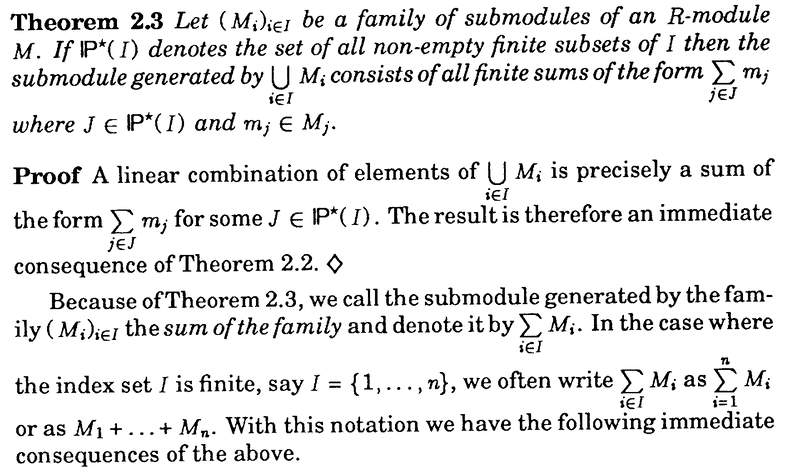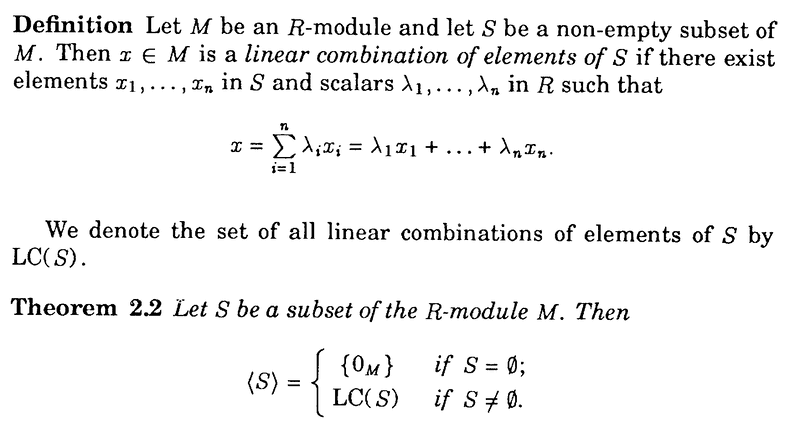# Generating modules and sub modules Blyth Theorem 2.3

Gold Member
I am reading T. S. Blyth's book: Module Theory: An Approach to Linear Algebra ...

I am focused on Chapter 2: Submodules; intersections and sums ... and need help with the proof of Theorem 2.3 ...In the above proof we read the following:

" ... ... A linear combination of elements of ##\bigcup_{ i \in I }## is precisely a sum of the form ##\sum_{ j \in J } m_j## for some ##J \in P(I)##. ... ... "

But ... Blyth defines a linear combination as in the text below ...So ... given the above definition wouldn't a linear combination of elements of ##\bigcup_{ i \in I } M_i## be a sum of the form ##\sum_{ j \in J } \lambda_j m_j## ... and not just ##\sum_{ j \in J } m_j## ... ... ?

Hope someone can help ...

Peter

#### Attachments

fresh_42
Mentor
2021 Award
The essential point of all such definitions is the finiteness of sums. All coefficients, resp. summands up to finitely many have to be zero. It can be different sets ##J \in \mathbb{P}^*(I)## from case to case, but in any sum there are still only finitely many.

As to your question: Theorem ##2.3## starts: Let ##(M_i)_{i\in I}## be a family of ##R-##submodules of an module ##M##. But as submodules, ##\lambda m = m'## is basically the same, i.e. it isn't necessary to carry the ##\lambda## all along the road, if the statement only requires ##m'##. The more, as we additionally have to bother the index sets: ##m=\sum_{i\in J_1}\lambda_i m_i\; , \;m'=\sum_{j \in J_2}\lambda'_j m_j\; , \;m+m'=\sum_{k \in J_1\cup J_2}(\lambda_k m_k+\lambda'_km'_k)## where ##\lambda_k=0## for ##k\in J_2-J_1## and ##\lambda'_k=0## for ##k\in J_1-J_2## ... etc. I understand, that it makes sense to drop all this unnecessary stuff and write ##m+m'=\sum_{k=1}^nm_k##.

If we talk about linear combinations, the ##\lambda## are necessary, because they are what makes it linear. If we talk about elements of modules, then ##\lambda_k m_k=m'_k\in M_k## both carry the same information, namely being elements of ##M_k##.

•Math Amateur
mathwonk
Homework Helper
Just repeating what fresh said essentially. I.e. Blyth didn't make clear quite what he meant. He is not changing the definition of linear combination. He meant that in this special case, where all the families Mi are themselves closed under scalar multiplication, then every linear combination involving scalars can be rewritten as a finite sum not involving scalars. I.e. as Fresh said, he could have been more clear if he had said, ":since in our case, for every scalar cj and element mj of Mj, the product cjmj = m'j is just another element m'j of Mj, every linear combination can be rewritten as just a finite sum of elements of the submodules Mj."

•Math Amateur
Gold Member
Just repeating what fresh said essentially. I.e. Blyth didn't make clear quite what he meant. He is not changing the definition of linear combination. He meant that in this special case, where all the families Mi are themselves closed under scalar multiplication, then every linear combination involving scalars can be rewritten as a finite sum not involving scalars. I.e. as Fresh said, he could have been more clear if he had said, ":since in our case, for every scalar cj and element mj of Mj, the product cjmj = m'j is just another element m'j of Mj, every linear combination can be rewritten as just a finite sum of elements of the submodules Mj."

Thanks to fresh_42 and mathwonk for clarifying this issue ...

Appreciate the help ...

Peter